# Sampling communities with mobsim

#### 2017-11-02

In addition to the simulation and analysis of spatially-explicit communities, `mobsim` provides a function to generate samples from simulated or observed communities. The combination of simulated data AND simulated sampling is a powerful approach to test the validity and power of empirical approaches.

# 1 Random sampling

Here, we simulate a community and then generate samples with the function `sample_quadrats`. By default `sample_quadrats` distributes a user-defined number of quadrats with user-defined size in the landscape and provides the number of individuals for each species in each quadrat.

The function returns two dataframes. The first includes the abundance of every species in every sampling quadrat and the second the positions of the lower left corners of the quadrats.

The community matrix of samples by species can then be analysed using additional software. For instance the R package vegan is perfectly suited for the analysis of community data. See the vignette Introduction to `mobsim` for a worked example.

``````library(mobsim)
sim_com1 <- sim_poisson_community(s_pool = 100, n_sim = 20000)``````
``sample1 <- sample_quadrats(sim_com1)``
``````## Warning in sample_quadrats(sim_com1): There are overlapping sampling
## squares in the design``````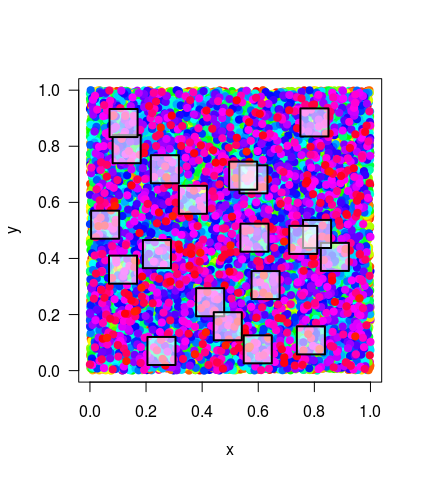``head(sample1\$spec_dat[,1:6])``
``````##       species1 species10 species100 species11 species12 species13
## site1       14         3          0         3         4         1
## site2       21         4          0         4         1         6
## site3       13         6          0         7         4         8
## site4       18         6          1         4         3         6
## site5       11         1          0         6         6         4
## site6       13         4          0         4         6         4``````
``head(sample1\$xy_dat)``
``````##                x         y
## site1 0.82308537 0.3555308
## site2 0.37847641 0.1938553
## site3 0.44134659 0.1084131
## site4 0.73764112 0.0579899
## site5 0.08093197 0.7395442
## site6 0.75966040 0.4373222``````

In `sample_quadrats()` there is an option to exclude overlapping quadrats from the random sampling design, which is shown here in two examples with different numbers and sizes of the quadrats.

``````sample2 <- sample_quadrats(sim_com1, n_quadrats= 2, quadrat_area = 0.1,
avoid_overlap = T)``````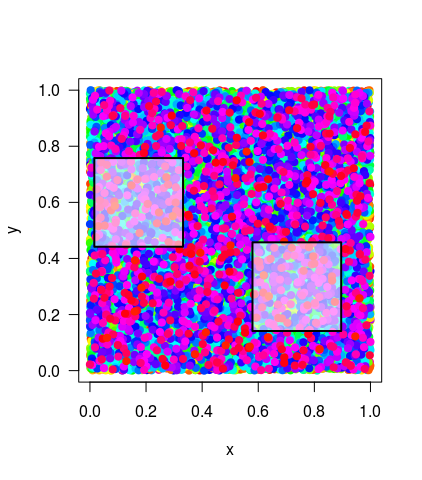``````sample3 <- sample_quadrats(sim_com1, n_quadrats= 20, quadrat_area = 0.001,
avoid_overlap = T)``````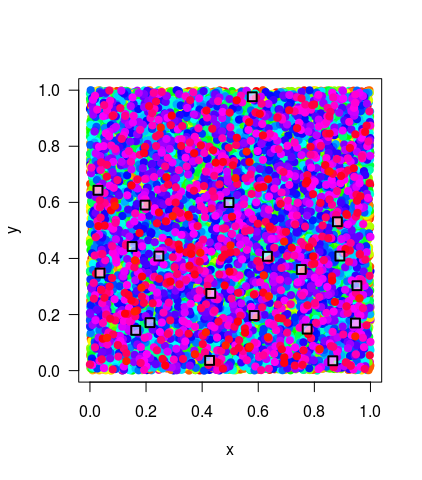# 2 Transect sampling

In addition to random designs also transects can be sampled-. This requires specifying a position for the lower left quadrat as well as x and y distances between neighbouring quadrats.

``````sample4 <- sample_quadrats(sim_com1, n_quadrats= 10, quadrat_area = 0.005,
method = "transect", x0 = 0, y0 = 0.5, delta_x = 0.1,
delta_y = 0)``````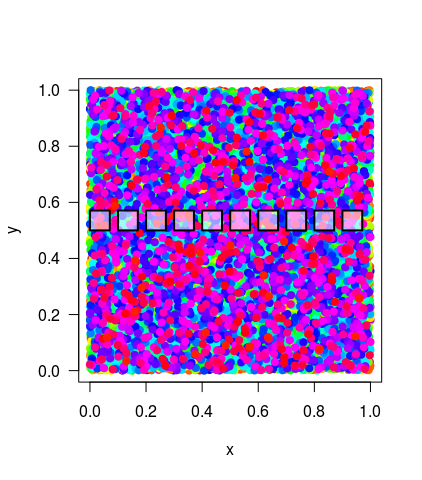``````sample5 <- sample_quadrats(sim_com1, n_quadrats= 10, quadrat_area = 0.005,
method = "transect", x0 = 0, y0 = 0, delta_x = 0.1,
delta_y = 0.1)``````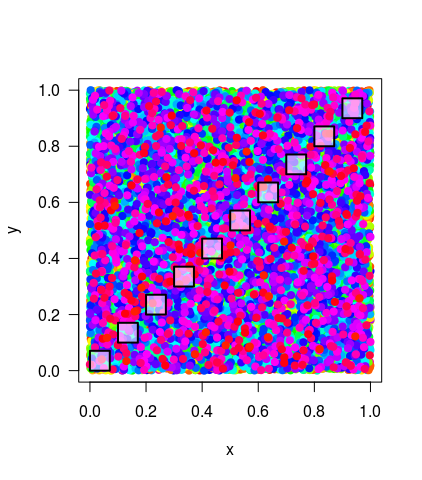# 3 Grid sampling

Finally, sampling quadrats can be arranged in a regular lattice. For this design users have to choose distances among the quadrats in x and y dimension as shown in the example.

``````sample6 <- sample_quadrats(sim_com1, n_quadrats= 25, quadrat_area = 0.005,
method = "grid", x0 = 0, y0 = 0, delta_x = 0.1,
delta_y = 0.1)``````